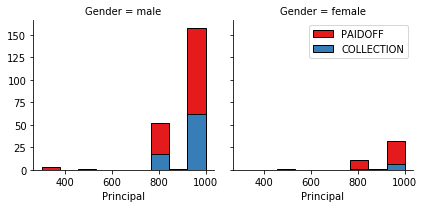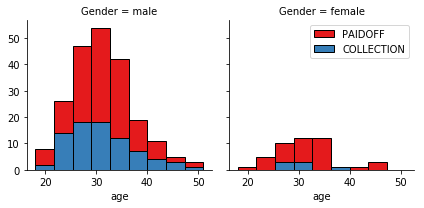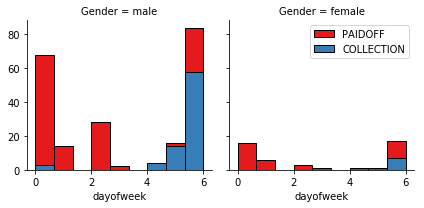# Machine Learning with Jupyter Labs

Posted by Daniel Vela on March 22, 2019# Classification with Python

In this notebook we try to practice all the classification algorithms that we learned in this course.

We load a dataset using Pandas library, and apply the following algorithms, and find the best one for this specific dataset by accuracy evaluation methods.

``````import itertools
import numpy as np
import matplotlib.pyplot as plt
from matplotlib.ticker import NullFormatter
import pandas as pd
import numpy as np
import matplotlib.ticker as ticker
from sklearn import preprocessing
%matplotlib inline
``````

This dataset is about past loans. The Loan_train.csv data set includes details of 346 customers whose loan are already paid off or defaulted. It includes following fields:

Field Description
Loan_status Whether a loan is paid off on in collection
Principal Basic principal loan amount at the
Terms Origination terms which can be weekly (7 days), biweekly, and monthly payoff schedule
Effective_date When the loan got originated and took effects
Due_date Since it’s one-time payoff schedule, each loan has one single due date
Age Age of applicant
Education Education of applicant
Gender The gender of applicant

``````!wget -O loan_train.csv https://s3-api.us-geo.objectstorage.softlayer.net/cf-courses-data/CognitiveClass/ML0101ENv3/labs/loan_train.csv
``````
``````--2019-03-12 10:06:53--  https://s3-api.us-geo.objectstorage.softlayer.net/cf-courses-data/CognitiveClass/ML0101ENv3/labs/loan_train.csv
Resolving s3-api.us-geo.objectstorage.softlayer.net (s3-api.us-geo.objectstorage.softlayer.net)... 67.228.254.193
Connecting to s3-api.us-geo.objectstorage.softlayer.net (s3-api.us-geo.objectstorage.softlayer.net)|67.228.254.193|:443... connected.
HTTP request sent, awaiting response... 200 OK
Length: 23101 (23K) [text/csv]
Saving to: ‘loan_train.csv’

100%[======================================>] 23,101      --.-K/s   in 0.002s

2019-03-12 10:06:53 (11.4 MB/s) - ‘loan_train.csv’ saved [23101/23101]
``````

### Load Data From CSV File

``````df = pd.read_csv('loan_train.csv')
``````
Unnamed: 0 Unnamed: 0.1 loan_status Principal terms effective_date due_date age education Gender
0 0 0 PAIDOFF 1000 30 9/8/2016 10/7/2016 45 High School or Below male
1 2 2 PAIDOFF 1000 30 9/8/2016 10/7/2016 33 Bechalor female
2 3 3 PAIDOFF 1000 15 9/8/2016 9/22/2016 27 college male
3 4 4 PAIDOFF 1000 30 9/9/2016 10/8/2016 28 college female
4 6 6 PAIDOFF 1000 30 9/9/2016 10/8/2016 29 college male
``````df.shape
``````
``````(346, 10)
``````

### Convert to date time object

``````df['due_date'] = pd.to_datetime(df['due_date'])
df['effective_date'] = pd.to_datetime(df['effective_date'])
``````
Unnamed: 0 Unnamed: 0.1 loan_status Principal terms effective_date due_date age education Gender
0 0 0 PAIDOFF 1000 30 2016-09-08 2016-10-07 45 High School or Below male
1 2 2 PAIDOFF 1000 30 2016-09-08 2016-10-07 33 Bechalor female
2 3 3 PAIDOFF 1000 15 2016-09-08 2016-09-22 27 college male
3 4 4 PAIDOFF 1000 30 2016-09-09 2016-10-08 28 college female
4 6 6 PAIDOFF 1000 30 2016-09-09 2016-10-08 29 college male

# Data visualization and pre-processing

Let’s see how many of each class is in our data set

``````df['loan_status'].value_counts()
``````
``````PAIDOFF       260
COLLECTION     86
Name: loan_status, dtype: int64
``````

260 people have paid off the loan on time while 86 have gone into collection

Lets plot some columns to underestand data better:

``````# notice: installing seaborn might takes a few minutes
!conda install -c anaconda seaborn -y
``````
``````Fetching package metadata .............
Solving package specifications: .

Package plan for installation in environment /opt/conda/envs/DSX-Python35:

The following packages will be UPDATED:

seaborn: 0.8.0-py35h15a2772_0 --> 0.9.0-py35_0 anaconda

seaborn-0.9.0- 100% |################################| Time: 0:00:00  47.36 MB/s
``````
``````import seaborn as sns

bins = np.linspace(df.Principal.min(), df.Principal.max(), 10)
g = sns.FacetGrid(df, col="Gender", hue="loan_status", palette="Set1", col_wrap=2)
g.map(plt.hist, 'Principal', bins=bins, ec="k")

g.axes[-1].legend()
plt.show()
````````````bins = np.linspace(df.age.min(), df.age.max(), 10)
g = sns.FacetGrid(df, col="Gender", hue="loan_status", palette="Set1", col_wrap=2)
g.map(plt.hist, 'age', bins=bins, ec="k")

g.axes[-1].legend()
plt.show()
``````# Pre-processing: Feature selection/extraction

### Lets look at the day of the week people get the loan

``````df['dayofweek'] = df['effective_date'].dt.dayofweek
bins = np.linspace(df.dayofweek.min(), df.dayofweek.max(), 10)
g = sns.FacetGrid(df, col="Gender", hue="loan_status", palette="Set1", col_wrap=2)
g.map(plt.hist, 'dayofweek', bins=bins, ec="k")
g.axes[-1].legend()
plt.show()

``````We see that people who get the loan at the end of the week dont pay it off, so lets use Feature binarization to set a threshold values less then day 4

``````df['weekend'] = df['dayofweek'].apply(lambda x: 1 if (x>3)  else 0)
``````
Unnamed: 0 Unnamed: 0.1 loan_status Principal terms effective_date due_date age education Gender dayofweek weekend
0 0 0 PAIDOFF 1000 30 2016-09-08 2016-10-07 45 High School or Below male 3 0
1 2 2 PAIDOFF 1000 30 2016-09-08 2016-10-07 33 Bechalor female 3 0
2 3 3 PAIDOFF 1000 15 2016-09-08 2016-09-22 27 college male 3 0
3 4 4 PAIDOFF 1000 30 2016-09-09 2016-10-08 28 college female 4 1
4 6 6 PAIDOFF 1000 30 2016-09-09 2016-10-08 29 college male 4 1

## Convert Categorical features to numerical values

Lets look at gender:

``````df.groupby(['Gender'])['loan_status'].value_counts(normalize=True)
``````
``````Gender  loan_status
female  PAIDOFF        0.865385
COLLECTION     0.134615
male    PAIDOFF        0.731293
COLLECTION     0.268707
Name: loan_status, dtype: float64
``````

86 % of female pay there loans while only 73 % of males pay there loan

Lets convert male to 0 and female to 1:

``````df['Gender'].replace(to_replace=['male','female'], value=[0,1],inplace=True)
``````
Unnamed: 0 Unnamed: 0.1 loan_status Principal terms effective_date due_date age education Gender dayofweek weekend
0 0 0 PAIDOFF 1000 30 2016-09-08 2016-10-07 45 High School or Below 0 3 0
1 2 2 PAIDOFF 1000 30 2016-09-08 2016-10-07 33 Bechalor 1 3 0
2 3 3 PAIDOFF 1000 15 2016-09-08 2016-09-22 27 college 0 3 0
3 4 4 PAIDOFF 1000 30 2016-09-09 2016-10-08 28 college 1 4 1
4 6 6 PAIDOFF 1000 30 2016-09-09 2016-10-08 29 college 0 4 1

## One Hot Encoding

``````df.groupby(['education'])['loan_status'].value_counts(normalize=True)
``````
``````education             loan_status
Bechalor              PAIDOFF        0.750000
COLLECTION     0.250000
High School or Below  PAIDOFF        0.741722
COLLECTION     0.258278
Master or Above       COLLECTION     0.500000
PAIDOFF        0.500000
college               PAIDOFF        0.765101
COLLECTION     0.234899
Name: loan_status, dtype: float64
``````

#### Feature befor One Hot Encoding

``````df[['Principal','terms','age','Gender','education']].head()
``````
Principal terms age Gender education
0 1000 30 45 0 High School or Below
1 1000 30 33 1 Bechalor
2 1000 15 27 0 college
3 1000 30 28 1 college
4 1000 30 29 0 college

#### Use one hot encoding technique to conver categorical varables to binary variables and append them to the feature Data Frame

``````Feature = df[['Principal','terms','age','Gender','weekend']]
Feature = pd.concat([Feature,pd.get_dummies(df['education'])], axis=1)
Feature.drop(['Master or Above'], axis = 1,inplace=True)

``````
Principal terms age Gender weekend Bechalor High School or Below college
0 1000 30 45 0 0 0 1 0
1 1000 30 33 1 0 1 0 0
2 1000 15 27 0 0 0 0 1
3 1000 30 28 1 1 0 0 1
4 1000 30 29 0 1 0 0 1

### Feature selection

Lets defind feature sets, X:

``````X = Feature
X[0:5]
``````
Principal terms age Gender weekend Bechalor High School or Below college
0 1000 30 45 0 0 0 1 0
1 1000 30 33 1 0 1 0 0
2 1000 15 27 0 0 0 0 1
3 1000 30 28 1 1 0 0 1
4 1000 30 29 0 1 0 0 1

What are our lables?

``````y = df['loan_status'].values
y[0:5]
``````
``````array(['PAIDOFF', 'PAIDOFF', 'PAIDOFF', 'PAIDOFF', 'PAIDOFF'], dtype=object)
``````

## Normalize Data

Data Standardization give data zero mean and unit variance (technically should be done after train test split )

``````X= preprocessing.StandardScaler().fit(X).transform(X)
X[0:5]
``````
``````array([[ 0.51578458,  0.92071769,  2.33152555, -0.42056004, -1.20577805,
-0.38170062,  1.13639374, -0.86968108],
[ 0.51578458,  0.92071769,  0.34170148,  2.37778177, -1.20577805,
2.61985426, -0.87997669, -0.86968108],
[ 0.51578458, -0.95911111, -0.65321055, -0.42056004, -1.20577805,
-0.38170062, -0.87997669,  1.14984679],
[ 0.51578458,  0.92071769, -0.48739188,  2.37778177,  0.82934003,
-0.38170062, -0.87997669,  1.14984679],
[ 0.51578458,  0.92071769, -0.3215732 , -0.42056004,  0.82934003,
-0.38170062, -0.87997669,  1.14984679]])
``````

# Classification

Now, it is your turn, use the training set to build an accurate model. Then use the test set to report the accuracy of the model You should use the following algorithm:

• K Nearest Neighbor(KNN)
• Decision Tree
• Support Vector Machine
• Logistic Regression

__ Notice:__

• You can go above and change the pre-processing, feature selection, feature-extraction, and so on, to make a better model.
• You should use either scikit-learn, Scipy or Numpy libraries for developing the classification algorithms.
• You should include the code of the algorithm in the following cells.

# K Nearest Neighbor(KNN)

Notice: You should find the best k to build the model with the best accuracy.
warning: You should not use the loan_test.csv for finding the best k, however, you can split your train_loan.csv into train and test to find the best k.

``````from sklearn.neighbors import KNeighborsClassifier
k = 6
#Train Model and Predict
model_knn = KNeighborsClassifier(n_neighbors = k)
model_knn.fit(X,y)
model_knn
``````
``````KNeighborsClassifier(algorithm='auto', leaf_size=30, metric='minkowski',
metric_params=None, n_jobs=1, n_neighbors=6, p=2,
weights='uniform')
``````
``````
``````
``````
``````

# Decision Tree

``````from sklearn.tree import DecisionTreeClassifier
model_tree = DecisionTreeClassifier(criterion="entropy", max_depth = 4)
model_tree.fit(X,y)
model_tree # it shows the default parameters
``````
``````DecisionTreeClassifier(class_weight=None, criterion='entropy', max_depth=4,
max_features=None, max_leaf_nodes=None,
min_impurity_decrease=0.0, min_impurity_split=None,
min_samples_leaf=1, min_samples_split=2,
min_weight_fraction_leaf=0.0, presort=False, random_state=None,
splitter='best')
``````
``````
``````
``````
``````

# Support Vector Machine

``````from sklearn import svm
model_svm = svm.SVC(kernel='linear')
model_svm.fit(X, y)
model_svm
``````
``````SVC(C=1.0, cache_size=200, class_weight=None, coef0=0.0,
decision_function_shape='ovr', degree=3, gamma='auto', kernel='linear',
max_iter=-1, probability=False, random_state=None, shrinking=True,
tol=0.001, verbose=False)
``````
``````
``````
``````
``````

# Logistic Regression

``````from sklearn.linear_model import LogisticRegression
model_lr = LogisticRegression(C=0.0001, solver='liblinear')
model_lr.fit(X,y)
model_lr
``````
``````LogisticRegression(C=0.0001, class_weight=None, dual=False,
fit_intercept=True, intercept_scaling=1, max_iter=100,
multi_class='ovr', n_jobs=1, penalty='l2', random_state=None,
solver='liblinear', tol=0.0001, verbose=0, warm_start=False)
``````
``````
``````
``````
``````

# Model Evaluation using Test set

``````from sklearn.metrics import jaccard_similarity_score
from sklearn.metrics import f1_score
from sklearn.metrics import log_loss
``````

``````!wget -O loan_test.csv https://s3-api.us-geo.objectstorage.softlayer.net/cf-courses-data/CognitiveClass/ML0101ENv3/labs/loan_test.csv
``````
``````--2019-03-12 11:19:01--  https://s3-api.us-geo.objectstorage.softlayer.net/cf-courses-data/CognitiveClass/ML0101ENv3/labs/loan_test.csv
Resolving s3-api.us-geo.objectstorage.softlayer.net (s3-api.us-geo.objectstorage.softlayer.net)... 67.228.254.193
Connecting to s3-api.us-geo.objectstorage.softlayer.net (s3-api.us-geo.objectstorage.softlayer.net)|67.228.254.193|:443... connected.
HTTP request sent, awaiting response... 200 OK
Length: 3642 (3.6K) [text/csv]
Saving to: ‘loan_test.csv’

100%[======================================>] 3,642       --.-K/s   in 0s

2019-03-12 11:19:01 (680 MB/s) - ‘loan_test.csv’ saved [3642/3642]
``````

### Load Test set for evaluation

``````test_df = pd.read_csv('loan_test.csv')
``````
Unnamed: 0 Unnamed: 0.1 loan_status Principal terms effective_date due_date age education Gender
0 1 1 PAIDOFF 1000 30 9/8/2016 10/7/2016 50 Bechalor female
1 5 5 PAIDOFF 300 7 9/9/2016 9/15/2016 35 Master or Above male
2 21 21 PAIDOFF 1000 30 9/10/2016 10/9/2016 43 High School or Below female
3 24 24 PAIDOFF 1000 30 9/10/2016 10/9/2016 26 college male
4 35 35 PAIDOFF 800 15 9/11/2016 9/25/2016 29 Bechalor male
``````# Prepare test set the same way the train set was evaluated
test_df['due_date'] = pd.to_datetime(test_df['due_date'])
test_df['effective_date'] = pd.to_datetime(test_df['effective_date'])
test_df['dayofweek'] = test_df['effective_date'].dt.dayofweek
test_df['weekend'] = test_df['dayofweek'].apply(lambda x: 1 if (x>3)  else 0)
test_df['Gender'].replace(to_replace=['male','female'], value=[0,1],inplace=True)

``````
Principal terms age Gender education
0 1000 30 50 1 Bechalor
1 300 7 35 0 Master or Above
2 1000 30 43 1 High School or Below
3 1000 30 26 0 college
4 800 15 29 0 Bechalor
``````Feature_test = test_df[['Principal','terms','age','Gender','weekend']]
Feature_test = pd.concat([Feature_test,pd.get_dummies(test_df['education'])], axis=1)
Feature_test.drop(['Master or Above'], axis = 1,inplace=True)
``````
Principal terms age Gender weekend Bechalor High School or Below college
0 1000 30 50 1 0 1 0 0
1 300 7 35 0 1 0 0 0
2 1000 30 43 1 1 0 1 0
3 1000 30 26 0 1 0 0 1
4 800 15 29 0 1 1 0 0
``````# Test data input
X_test = Feature_test
X_test = preprocessing.StandardScaler().fit(X_test).transform(X_test)
X_test[0:5]
``````
``````# Test data output
from sklearn import metrics
y_test = test_df['loan_status'].values
y_test[0:5]
``````
``````array(['PAIDOFF', 'PAIDOFF', 'PAIDOFF', 'PAIDOFF', 'PAIDOFF'], dtype=object)
``````
``````# ACCURACY SCORES

# knn
yhat = model_knn.predict(X_test)
yhat
print("Train set KNN Accuracy: ", metrics.accuracy_score(y, model_knn.predict(X)))
print("Test set KNN Accuracy: ", metrics.accuracy_score(y_test, yhat))
knn_jaccard = jaccard_similarity_score(y_test, yhat)
knn_f1_score = f1_score(y_test, yhat, average='weighted')

# Decission tree
yhat = model_tree.predict(X_test)
yhat
print("Train set Decission Tree Accuracy: ", metrics.accuracy_score(y, model_tree.predict(X)))
print("Test set Decission Tree Accuracy: ", metrics.accuracy_score(y_test, yhat))
tree_jaccard = jaccard_similarity_score(y_test, yhat)
tree_f1_score = f1_score(y_test, yhat, average='weighted')

# SVM
yhat = model_svm.predict(X_test)
yhat
print("Train set SVM Accuracy: ", metrics.accuracy_score(y, model_svm.predict(X)))
print("Test set SVM Accuracy: ", metrics.accuracy_score(y_test, yhat))
svm_jaccard = jaccard_similarity_score(y_test, yhat)
svm_f1_score = f1_score(y_test, yhat, average='weighted')

# Logistic regression
yhat = model_lr.predict(X_test)
yhat_proba = model_lr.predict_proba(X_test)
yhat
print("Train set Logistic regression Accuracy: ", metrics.accuracy_score(y, model_lr.predict(X)))
print("Test set Logistic regression Accuracy: ", metrics.accuracy_score(y_test, yhat))
lr_jaccard = jaccard_similarity_score(y_test, yhat)
lr_f1_score = f1_score(y_test, yhat, average='weighted')
lr_log_loss = log_loss(y_test, yhat_proba)
``````
``````Train set KNN Accuracy:  0.806358381503
Test set KNN Accuracy:  0.685185185185
Train set Decission Tree Accuracy:  0.751445086705
Test set Decission Tree Accuracy:  0.777777777778
Train set SVM Accuracy:  0.751445086705
Test set SVM Accuracy:  0.740740740741
Train set Logistic regression Accuracy:  0.745664739884
Test set Logistic regression Accuracy:  0.759259259259

/opt/conda/envs/DSX-Python35/lib/python3.5/site-packages/sklearn/metrics/classification.py:1135: UndefinedMetricWarning: F-score is ill-defined and being set to 0.0 in labels with no predicted samples.
'precision', 'predicted', average, warn_for)
``````
``````report = pd.DataFrame(data=np.array([["KNN", knn_jaccard, knn_f1_score, np.nan],
["Decision Tree", tree_jaccard, tree_f1_score, np.nan],
["SVM", svm_jaccard, svm_f1_score, np.nan],
["LogisticRegression", lr_jaccard, lr_f1_score, lr_log_loss]]), columns=["Algorithm", "Jaccard", "F1-score", "LogLoss"])
report = report.set_index(["Algorithm", "Jaccard", "F1-score", "LogLoss"])
# Jaccard
report
``````
Algorithm Jaccard F1-score LogLoss
KNN 0.685185185185 0.681298582533 nan
Decision Tree 0.777777777778 0.728395061728 nan
SVM 0.740740740741 0.630417651694 nan
LogisticRegression 0.759259259259 0.671764237356 0.689904024181